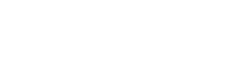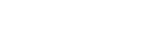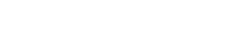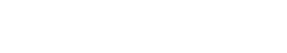## 2、推导## 3、梯度下降算法变种MBGD是训练神经网络最常用的优化方法。

## 4、挑战

1） 如何选择合适的学习率？

2） 如何确定学习率的调整策略？

3） 对所有参数的更新采用相同的学习率是否恰当？

4） 如何跳出局部最优？

Momentum

RMSprop

## 1、批量梯度下降(BGD)

```#引库
#引入matplotlib库,用于画图
import matplotlib.pyplot as plt
from math import pow
#图片嵌入jupyter
%matplotlib inline
#为了便于取用数据,我们将数据分为x,y,在直角坐标系中(x,y)是点
x = [1,2,3,4,5,6]
y = [13,14,20,21,25,30]
print("打印初始数据图...")
plt.scatter(x,y)
plt.xlabel("X")
plt.ylabel("Y")
plt.show()
#超参数设定
alpha = 0.01#学习率/步长
theta0 = 0#θ0
theta1 = 0#θ1
epsilon = 0.001#误差
m = len(x)
count = 0
loss = []
for time in range(1000):
count += 1
#求偏导theta0和theta1的结果
temp0 = 0#J(θ)对θ0求导的结果
temp1 = 0#J(θ)对θ1求导的结果
diss = 0
for i in range(m):
temp0 += (theta0+theta1*x[i]-y[i])/m
temp1 += ((theta0+theta1*x[i]-y[i])/m)*x[i]
#更新theta0和theta1
for i in range(m):
theta0 = theta0 - alpha*((theta0+theta1*x[i]-y[i])/m)
theta1 = theta1 - alpha*((theta0+theta1*x[i]-y[i])/m)*x[i]
#求损失函数J(θ)
for i in range(m):
diss = diss + 0.5*(1/m)*pow((theta0+theta1*x[i]-y[i]),2)
loss.append(diss)
#看是否满足条件
'''
if diss<=epsilon:
break
else:
continue
'''
print("最终的结果为:")
print("此次迭代次数为:{}次,最终theta0的结果为:{},最终theta1的结果为:{}".format(count,theta0,theta1))
print("预测的最终回归函数为:y={}+{}x\n".format(theta0,theta1))
print("迭代图像绘制...")
plt.scatter(range(count),loss)
plt.show()```## 2、随机梯度下降(SGD)

```#引库
#引入matplotlib库,用于画图
import matplotlib.pyplot as plt
from math import pow
import numpy as np
#图片嵌入jupyter
%matplotlib inline
#为了便于取用数据,我们将数据分为x,y,在直角坐标系中(x,y)是点
x = [1,2,3,4,5,6]
y = [13,14,20,21,25,30]
print("打印初始数据图...")
plt.scatter(x,y)
plt.xlabel("X")
plt.ylabel("Y")
plt.show()
#超参数设定
alpha = 0.01#学习率/步长
theta0 = 0#θ0
theta1 = 0#θ1
epsilon = 0.001#误差
m = len(x)
count = 0
loss = []
for time in range(1000):
count += 1
diss = 0
#求偏导theta0和theta1的结果
temp0 = 0#J(θ)对θ0求导的结果
temp1 = 0#J(θ)对θ1求导的结果
for i in range(m):
temp0 += (theta0+theta1*x[i]-y[i])/m
temp1 += ((theta0+theta1*x[i]-y[i])/m)*x[i]
#更新theta0和theta1
for i in range(m):
theta0 = theta0 - alpha*((theta0+theta1*x[i]-y[i])/m)
theta1 = theta1 - alpha*((theta0+theta1*x[i]-y[i])/m)*x[i]
#求损失函数J(θ)
rand_i = np.random.randint(0,m)
diss += 0.5*(1/m)*pow((theta0+theta1*x[rand_i]-y[rand_i]),2)
loss.append(diss)
#看是否满足条件
'''
if diss<=epsilon:
break
else:
continue
'''
print("最终的结果为:")
print("此次迭代次数为:{}次,最终theta0的结果为:{},最终theta1的结果为:{}".format(count,theta0,theta1))
print("预测的最终回归函数为:y={}+{}x\n".format(theta0,theta1))
print("迭代图像绘制...")
plt.scatter(range(count),loss)
plt.show()```## 3、小批量梯度下降(MBGD)

```#引库
#引入matplotlib库,用于画图
import matplotlib.pyplot as plt
from math import pow
import numpy as np
#图片嵌入jupyter
%matplotlib inline
#为了便于取用数据,我们将数据分为x,y,在直角坐标系中(x,y)是点
x = [1,2,3,4,5,6]
y = [13,14,20,21,25,30]
print("打印初始数据图...")
plt.scatter(x,y)
plt.xlabel("X")
plt.ylabel("Y")
plt.show()
#超参数设定
alpha = 0.01#学习率/步长
theta0 = 0#θ0
theta1 = 0#θ1
epsilon = 0.001#误差
diss = 0#损失函数
m = len(x)
count = 0
loss = []
for time in range(1000):
count += 1
diss = 0
#求偏导theta0和theta1的结果
temp0 = 0#J(θ)对θ0求导的结果
temp1 = 0#J(θ)对θ1求导的结果
for i in range(m):
temp0 += (theta0+theta1*x[i]-y[i])/m
temp1 += ((theta0+theta1*x[i]-y[i])/m)*x[i]
#更新theta0和theta1
for i in range(m):
theta0 = theta0 - alpha*((theta0+theta1*x[i]-y[i])/m)
theta1 = theta1 - alpha*((theta0+theta1*x[i]-y[i])/m)*x[i]
#求损失函数J(θ)
result = []
for i in range(3):
rand_i = np.random.randint(0,m)
result.append(rand_i)
for j in result:
diss += 0.5*(1/m)*pow((theta0+theta1*x[j]-y[j]),2)
loss.append(diss)
#看是否满足条件
'''
if diss<=epsilon:
break
else:
continue
'''
print("最终的结果为:")
print("此次迭代次数为:{}次,最终theta0的结果为:{},最终theta1的结果为:{}".format(count,theta0,theta1))
print("预测的最终回归函数为:y={}+{}x\n".format(theta0,theta1))
print("迭代图像绘制...")
plt.scatter(range(count),loss)
plt.show()```